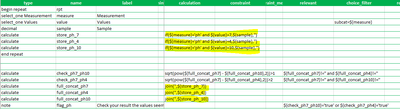# Extract values from repeat for calculation

283
2
04-08-2022 10:35 AMby
New Contributor II

Hello,

I am trying to save a set of specific values from a repeat in order to do some validation.

To simplify here is what I want to do :

Repeat 1 :

• Question 1 = ph
• Question 2 = 7
• Question 3 = 20

Repeat 2 :

• Question 1 = orp
• Question 2 = 124
• Question 3 = 5

Repeat 3 :

• Question 1 = ph
• Question 2 = 4
• Question 3 = 15

Outside of the repeat I want to store the Q3 value in one variable (call it store_ph_7) if Q1 = ph and Q2 = 7 and in another variable (call it store_ph_4) store the Q3 value for Q1 = ph and Q2 = 4

Then I have a note popping up if X - Y > 1

Is there a way to do this? Repeats will never be in the same order and the actual form have way more options so I can't rely on counts or indexes.

I based my structure on the assumption that I could store any value from the repeat using a set of relevant rules but apparently it doesn't work that way. I tried using once() but that didn't work either.

Anyone has a clever trick to do this?

2 Repliesby
New Contributor II

I found two work arounds, and implemented one. But please let me know if there is something easier!

One solution is using concat in the repeat to create a json like structure to store all the data. Then using join outside of the repeat to make a list of json like object contain, each item containing the data for one repeat. Which in turn can be parsed using a custom javascript function... Messy and dirty but it works.

The second solution I have found, which only works because I have only three values to store is using join. Using an if in my calculation within the repeat, I insure that only one value will be returned for all the repeats iteration. The join outside of the repeat will then only have one value to join and will have stored the data I need. Here is an image showing the mess :Not pretty but it works, I am sure there are better way to do this so let me know 😄byEsri Contributor

Hi

From Ismael's blog :

When you pass a question within a repeat to pulldata("@javascript"), the JavaScript function receives an array of values for the specified question. For example, lets say we want to display a warning message if the user has introduced duplicate values in a question within a repeat. In this case, we want to create a JavaScript function that takes an array and returns true if duplicate values are found. Something like this:

function HasDups (myArray)
{
return new Set(myArray).size !== myArray.length;
}

Regards